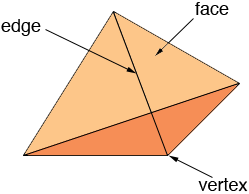SEARCH HOMEMath Central Quandaries & QueriesQuestion from shani, a student: my homework question is confusing (yr 7 beginner) "How many vertices does a triangular pyramid have." (Mum thinks all triangles are triangular & vertices are sides) I want to be sure.Hi Shani,

A triangular pyramid is made from 4 triangles. These triangles are called the faces of the pyramid, the lines that form the borders of the triangles are called the edges of the pyramid and the vertices are the points where the edges meet.I hope this helps,
PennyMath Central is supported by the University of Regina and The Pacific Institute for the Mathematical Sciences.